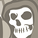# New to Qlik Sense

If you’re new to Qlik Sense, start with this Discussion Board and get up-to-speed quickly.

Announcements
QlikWorld Online 2021, May 10-12: Our Free, Virtual, Global Event REGISTER TODAY
cancel
Showing results for
Search instead for
Did you mean:Creator

## If statment to Set analysis

Hi Qlikers,

I have the below if statement and it work fine on table but I need it to be working as KPI (Set Analysis).

if(
(sum({<MMT_DATE={">=\$(=date(num((Max(As_At_Date)))))<=\$(=date(num((Max(As_At_Date_2)))))"}>}distinct LN_QTY)
>
Sum({<MMT_DATE={">=\$(=date(num((Max(As_At_Date)))))<=\$(=date(num((Max(As_At_Date_2)))))"}>} MMT_QUANTITY)),

Count(distinct TRANSACTION_SOURCE_ID_1)
)

does any have idea how we can convert it into a set analysis or even another solution to it .

Thanks In Advance.
Labels (1)
• ### if&setanalysis

1 Solution

Accepted SolutionsMVP

May bad, try this (misplaced ] and "... fixed it now)

``````Count(DISTINCT

{<TRANSACTION_SOURCE_ID_1 = {"=Sum({<MMT_DATE = {[>=\$(=Date(Max(As_At_Date)))<=\$(=Date(Max(As_At_Date_2)))]}>} DISTINCT LN_QTY) > Sum({<MMT_DATE={[>=\$(=Date(Max(As_At_Date)))<=\$(=Date(Max(As_At_Date_2)))]}>} MMT_QUANTITY)"}>}

TRANSACTION_SOURCE_ID_1)``````

9 RepliesMVP

May be this

``````Count(DISTINCT

{<TRANSACTION_SOURCE_ID_1 = {"=Sum({<MMT_DATE = {[>=\$(=Date(Max(As_At_Date)))<=\$(=Date(Max(As_At_Date_2)))]}>} DISTINCT LN_QTY) > Sum({<MMT_DATE={[>=\$(=Date(Max(As_At_Date)))<=\$(=Date(Max(As_At_Date_2)))"}>} MMT_QUANTITY)]"}>}

TRANSACTION_SOURCE_ID_1)``````Creator
Author

@sunny_talwar  thanks for your repley Sunny.

but it still gives me an error  cause of the brackets [] not sure if it should be a part of the set analysis or no !!MVP

May bad, try this (misplaced ] and "... fixed it now)

``````Count(DISTINCT

{<TRANSACTION_SOURCE_ID_1 = {"=Sum({<MMT_DATE = {[>=\$(=Date(Max(As_At_Date)))<=\$(=Date(Max(As_At_Date_2)))]}>} DISTINCT LN_QTY) > Sum({<MMT_DATE={[>=\$(=Date(Max(As_At_Date)))<=\$(=Date(Max(As_At_Date_2)))]}>} MMT_QUANTITY)"}>}

TRANSACTION_SOURCE_ID_1)``````Partner

still brackets instead of double quotes....

Count(DISTINCT

{<TRANSACTION_SOURCE_ID_1 = {"=Sum({<MMT_DATE = {">=\$(=Date(Max(As_At_Date)))<=\$(=Date(Max(As_At_Date_2)))"}>} DISTINCT LN_QTY) > Sum({<MMT_DATE={">=\$(=Date(Max(As_At_Date)))<=\$(=Date(Max(As_At_Date_2)))"}>} MMT_QUANTITY)"}>}

TRANSACTION_SOURCE_ID_1)MVP

Yes because double quotes within double quotes won't work unless you use an escape sequence

``````Count(DISTINCT

{<TRANSACTION_SOURCE_ID_1 = {"=Sum({<MMT_DATE = {"">=\$(=Date(Max(As_At_Date)))<=\$(=Date(Max(As_At_Date_2)))""}>} DISTINCT LN_QTY) > Sum({<MMT_DATE={"">=\$(=Date(Max(As_At_Date)))<=\$(=Date(Max(As_At_Date_2)))""}>} MMT_QUANTITY)"}>}

TRANSACTION_SOURCE_ID_1)``````

or use square brackets like mentioned before

``````Count(DISTINCT

{<TRANSACTION_SOURCE_ID_1 = {"=Sum({<MMT_DATE = {[>=\$(=Date(Max(As_At_Date)))<=\$(=Date(Max(As_At_Date_2)))]}>} DISTINCT LN_QTY) > Sum({<MMT_DATE={[>=\$(=Date(Max(As_At_Date)))<=\$(=Date(Max(As_At_Date_2)))"}>} MMT_QUANTITY)]}>}

TRANSACTION_SOURCE_ID_1)``````Partner

Really? You can escape double quotes using brackets?

Can you also escape single quotes this way (for strings inside dynamic expressions)?Creator
Author

@sunny_talwar

many thanks Sunny for sharing your knowledge....

Rock Star like always 🙂MVP

I am not really sure if [] is equivalent of single quotes or double quotes, but we know that single quotes and double quotes are interchangeable in some cases.... in those cases, I would assume that single quote or double quote can be replaced with square brackets.MVP

No problem at all, I am always happy to help.